# WASSCE / WAEC Further Maths Syllabus

## WASSCE / WAEC Further Maths Syllabus

FURTHER MATHEMATICS/MATHEMATICS (ELECTIVE)

AIMS OF THE SYLLABUS

The aims of the syllabus are to test candidates’

(i)development of further conceptual and manipulative skills in Mathematics;

(ii)understanding of an intermediate course of study which bridges the gap between Elementary Mathematics and Higher Mathematics;

(iii)acquisition of aspects of Mathematics that can meet the needs of potential Mathematicians, Engineers, Scientists and other professionals.

(iv)ability to analyse data and draw valid conclusion

(v)logical, abstract and precise reasoning skills.

EXAMINATION SCHEME

There will be two papers, Papers 1 and 2, both of which must be taken.

PAPER 1: will consist of forty multiple-choice objective questions, covering the entire syllabus. Candidates will be required to answer all questions in 1hours for 40 marks. The questions will be drawn from the sections of the syllabus as follows:

 Pure Mathematics – 30 questions Statistics and probability – 4 questions Vectors and Mechanics – 6 questions

PAPER 2: will consist of two sections, Sections A and B, to be answered in 2hours for 100 marks.

Section A will consist of eight compulsory questions that areelementary in type for 48 marks. The questions shall be distributed as follows:

 Pure Mathematics – 4 questions Statistics and Probability – 2 questions Vectors and Mechanics – 2 questions

Section B will consist of seven questions of greater length and difficulty put into three parts:Parts I, II and III as follows:

 Part I: Pure Mathematics – 3 questions

WAEC Syllabus – Uploaded online by www.myschoolgist.com.ng

 Part II: Statistics and Probability – 2 questions Part III: Vectors and Mechanics – 2 questions

Candidates will be required to answer four questions with at least one from each part for 52 marks.

DETAILED SYLLABUS

In addition to the following topics, more challenging questions may be set on topics in the General Mathematics/Mathematics (Core) syllabus.

In the column for CONTENTS, more detailed information on the topics to be tested is given while the limits imposed on the topics are stated under NOTES.

Topics which are marked with asterisks shall be tested in Section B of Paper 2 only.

KEY:

*Topics peculiar to Ghana only. ** Topics peculiar to Nigeria only

 Topics Content Notes I. Pure Mathematics (1) Sets (i) Idea of a set defined by a (x : x is real), ∪, ∩, { },∉, ∈, property, Set notations and their ⊂, ⊆, meanings. (ii) Disjoint sets, Universal set and U (universal set) and complement of set A’ (Complement of set A). (iii) Venn diagrams, Use of sets More challenging problems And Venn diagrams to solve involving union, intersection, problems. the universal set, subset and complement of set. (iv) Commutative and Associative Three set problems. Use of De laws, Distributive properties Morgan’s laws to solve related over union and intersection. problems  (2) Surds √ All the four operations on surds Surds of the form √ , a and Rationalising the denominator a+b√ where a is rational, b is a  of surds such as ,  √ , positive integer and n is not a √ √ perfect square.

WAEC Syllabus – Uploaded online by www.myschoolgist.com.ng

 (3) Binary Operations Properties: Closure, Commutativity, Associativity and Distributivity, Identity elements and inverses. (4) Logical Reasoning (i) Rule of syntax: true or false statements, rule of logic applied to arguments, implications and deductions. (ii) The truth table (5) Functions (i) Domain and co-domain of a function. (ii) One-to-one, onto, identity and constant mapping; (iii) Inverse of a function. (iv) Composite of functions. (6) Polynomial (i) Linear Functions, Equations and Functions Inequality

 .

Use of properties to solve related problems.

Using logical reasoning to determine the validity of compound statements involving implications and connectivities. Include use of symbols: ~P

ν q, p ∧ q, p ⇒ q

Use of Truth tables to deduce conclusions of compound statements. Include negation.

The notation e.g. f : x 3x+4;

g: x → x; where x R.

Graphical representation of a function ; Image and the range.

Determination of the inverse of a one-to-one function e.g. If f: x sx + , the inverse

relation f-1: x x – is also a function.

Notation: fog(x) =f(g(x)) Restrict to simple algebraic functions only.

Recognition and sketching of graphs of linear functions and equations.

of linear equations i.e.

ax + by + c = 0; y = mx + c; = k. Parallel and

Perpendicular lines. Linear Inequalities e.g. 2x + 5y ≤ 1, x + 3y ≥ 3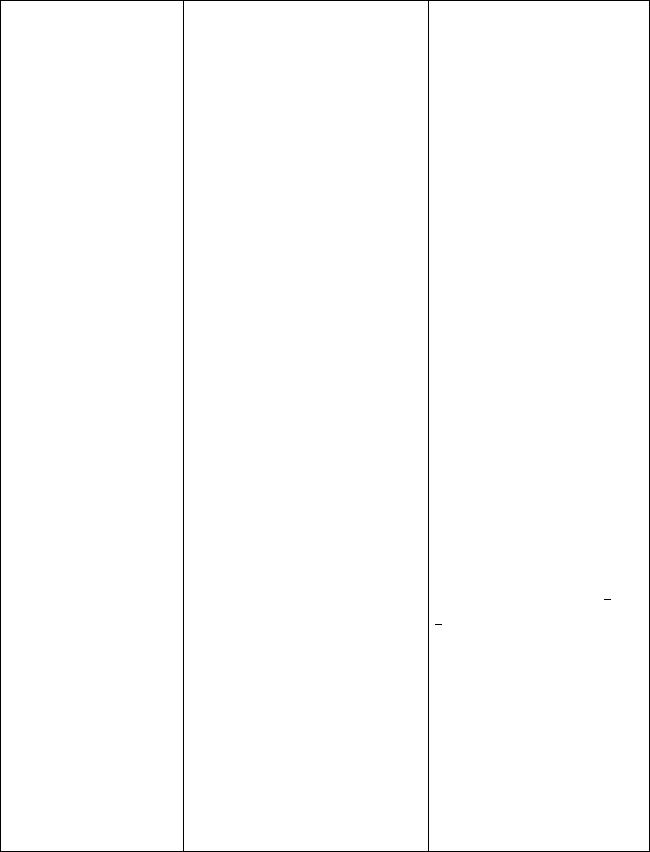Graphical representation of linear inequalities in two variables. Application to Linear Programming.
Recognition and sketching graphs of quadratic functions e.g.
f: x → ax+bx + c, where a, b and c Є R.
Identification of vertex, axis of symmetry, maximum and minimum, increasing and decreasing parts of a parabola. Include values of x for which f(x) >0 or f(x) < 0.
Solution of simultaneous equations: one linear and one quadratic. Method of completing the squares for solving quadratic equations. Express f(x) = ax+ bx + c in the form f(x) = a(x + d)+ k, where k is the maximum or minimum value. Roots of quadratic equations – equal roots (b– 4ac = 0), real and unequal roots (b– 4ac > 0), imaginary roots (b– 4ac < 0); sum and product of roots of a quadratic equation e.g. if the roots of the equation 3x2+ 5x + 2 = 0 are and β, form the
equation whose roots areand . Solving quadratic inequalities.
Recognition of cubic functions e.g. f: x → ax+ bx+cx + d. Drawing graphs of cubic functions for a given range. Factorization of cubic expressions and solution of cubic equations. Factorization of a± b3. Basic operations on polynomials, the remainder and factor theorems i.e. the

WAEC Syllabus – Uploaded online by www.myschoolgist.com.ng

and Inequalities

(ii) Cubic Functions and Equations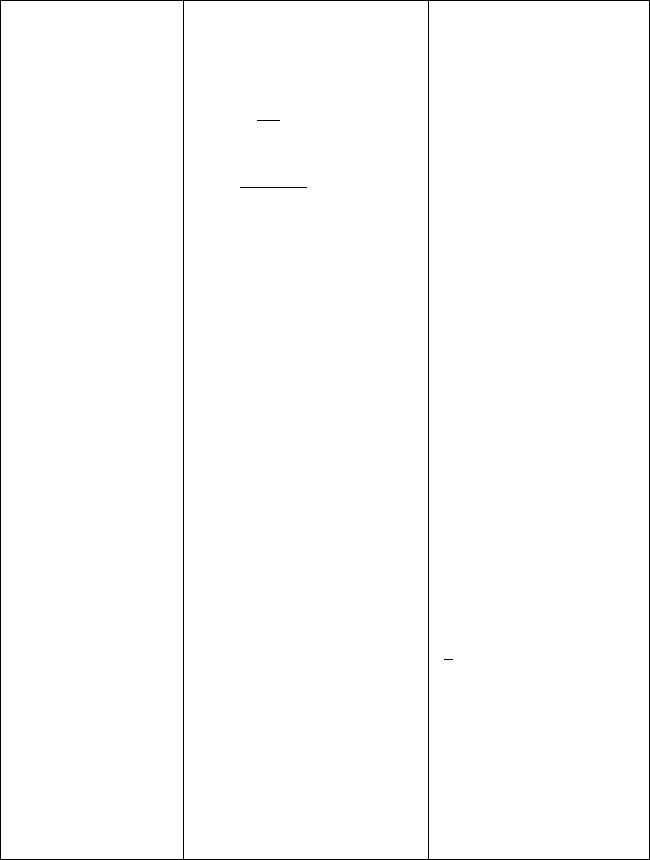WAEC Syllabus – Uploaded online by www.myschoolgist.com.ng

(7) Rational Functions

(8)Indices and Logarithmic Functions

(i) Rational functions of the form Q(x) = !()() ,g(x) ≠ 0.

where g(x) and f(x) are

polynomials. e.g.

f:x “#\$%

(ii)Resolution of rational functions into partial

fractions.

(i)Indices

(ii)Logarithms

remainder when f(x) is divided by f(x – a) = f(a). When f(a) is zero, then (x – a) is a factor of f(x).

g(x) may be factorised into linear and quadratic factors (Degree of Numerator less than that of denominator which is less than or equal to 4).

The four basic operations. Zeros, domain and range, sketching not required.

Laws of indices. Application of the laws of indices to evaluating products, quotients, powers and nth root. Solve equations involving indices.

Laws of Logarithms. Application of logarithms in calculations involving product, quotients, power (log an), nth roots (log √&, log a1/n).

Solve equations involving logarithms (including change of base).

Reduction of a relation such as y = axb, (a,b are constants) to a linear form:

log10y = b log10x+log10a. Consider other examples such

as

log ab= log a + x log b;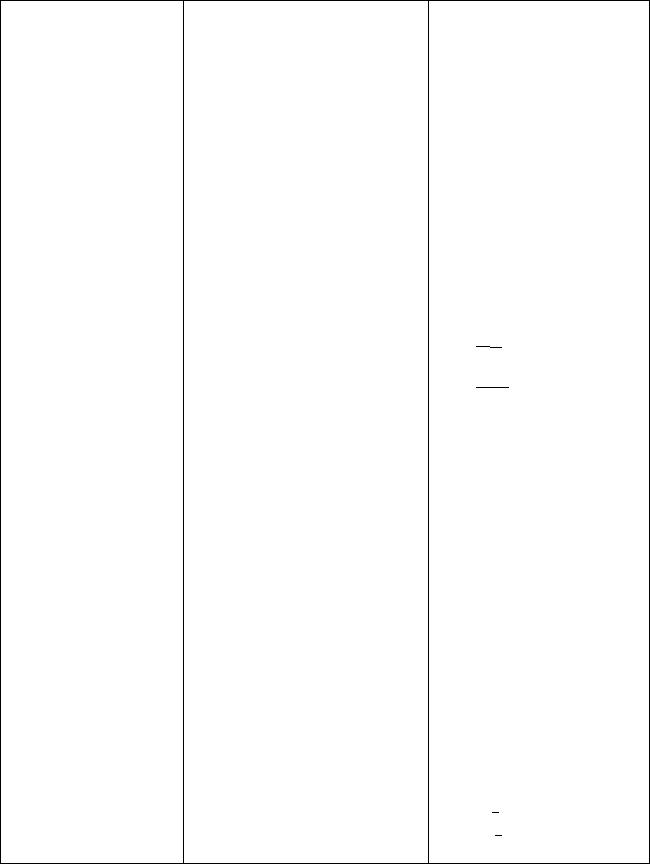WAEC Syllabus – Uploaded online by www.myschoolgist.com.ng

(9) Permutation And Combinations.

(10)Binomial Theorem

(11)Sequences and Series

(i)Simple cases of arrangements

(ii)Simple cases of selection of objects.

Expansion of (a + b)n.

Use of (1+x)≈1+nx for any rational n, where x is sufficiently small. e.g (0.998)1/3

(i)Finite and Infinite sequences.

(ii)Linear sequence/Arithmetic Progression (A.P.) and

Exponential sequence/Geometric

Progression (G.P.)

(iii)Finite and Infinite series.

(iv)Linear series (sum of A.P.) and exponential series (sum of G.P.)

log (ab)= x(log a + log b)

=x log ab

*Drawing and interpreting

graphs of logarithmic functions e.g. y = axb. Estimating the values of the constants a and b from the graph

Knowledge of arrangement and selection is expected. The notations: nCr(%and nPfor selection and arrangement respectively should be noted and used. e.g. arrangement of students in a row, drawing balls from a box with or without

replacements. np= n!

(n-r)!

nCr= n! r!(n-r)!

Use of the binomial theorem for positive integral index only. Proof of the theorem not required.

e.g. (i) u1, u2,…, un.(ii) u1, u2,….

Recognizing the pattern of a sequence. e.g.

(i)U= U+ (n-1)d, where d is the common difference.

(ii)Un= Urn-1 where r is the common ratio.

(i)U+ U+ U+ … + Un(ii)U+ U+ U+ ….

(i)S((U1+Un)

(ii)S([2a + (n – 1)d]

WAEC Syllabus – Uploaded online by www.myschoolgist.com.ng

*(v) Recurrence Series

 (12)Matrices and (i) Matrices Linear Transformation

(ii) Determinants

(iii)Inverse of 2 x 2 Matrices

(iv)Linear Transformation

(iii)Sn= U1(1-rn) , r<1

l – r

(iv)Sn=U1(rn-1) , r>l. r – 1

(v)Sum to infinity (S) =%r < 1

Generating the terms of a recurrence series and finding an explicit formula for the sequence e.g. 0.9999 =

****+ ….

Concept of a matrix – state the order of a matrix and indicate the type.

Equal matrices – If two matrices are equal, then their corresponding elements are equal. Use of equality to find missing entries of given matrices

Addition and subtraction of matrices (up to 3 x 3 matrices). Multiplication of a matrix by a scalar and by a matrix (up to 3 x 3 matrices)

Evaluation of determinants of 2 x 2 matrices.

**Evaluation of determinants of 3 x 3 matrices.

Application of determinants to solution of simultaneous linear equations.

 e.g. If A = &  , then – . − -1  . A = &   −-

Finding the images of points under given linear transformation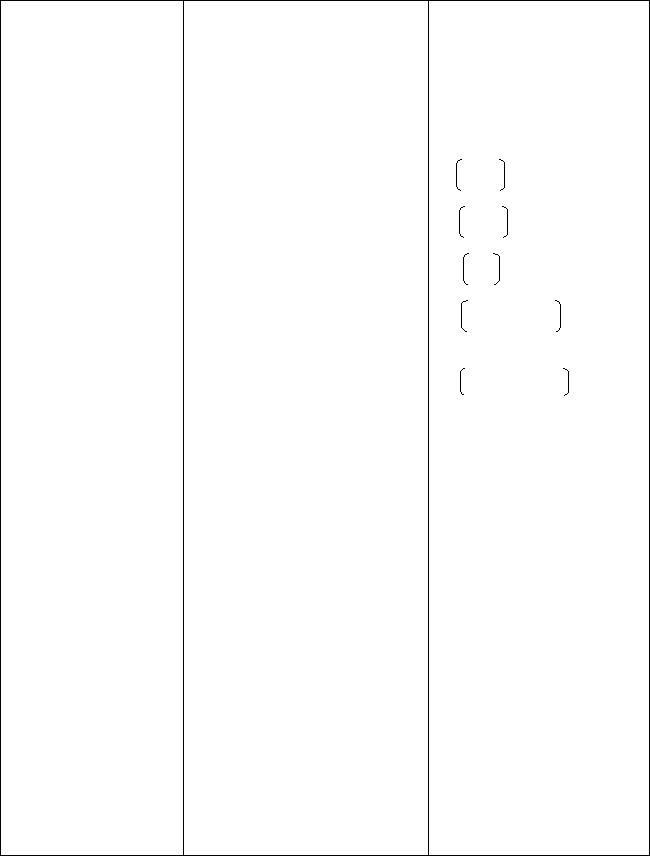WAEC Syllabus – Uploaded online by www.myschoolgist.com.ng

Determining the matrices of given linear transformation. Finding the inverse of a linear transformation (restrict to 2 x 2 matrices).

Finding the composition of linear transformation. Recognizing the Identity transformation.

 (i) 1 0 reflection in the 0 −1 x – axis (ii) −1 0 reflection in the 0 1 y – axis (iii) 0 1 reflection in the line 1 0 y = x (iv) cos 5 − sin 5for anti- sin 5 cos 5 clockwise rotation through θ about the origin. (v) -8925 sin 25 , the 9;25 −-8925 general matrix for reflection in a line through the origin making an angle θ with the positive x-axis. *Finding the equation of the image of a line under a given linear transformation (13)Trigonometry (i) Trigonometric Ratios and Rules Sine, Cosine and Tangent of general angles (0o≤θ≤360o). Identify trigonometric ratios of angles 30O, 45O, 60o without use of tables. Use basic trigonometric ratios and reciprocals to prove given trigonometric identities. Evaluate sine, cosine and tangent of negative angles. Convert degrees into radians and vice versa. Application to real life situations such as heights and distances, perimeters, solution of triangles, angles of elevation and depression,WAEC Syllabus – Uploaded online by www.myschoolgist.com.ng

 bearing(negative and positive angles) including use of sine and cosine rules, etc, Simple cases only. (ii) Compound and Multiple Angles. sin (A± B),cos (A ± B), tan(A ± B). Use of compound angles in simple identities and solution of trigonometric ratios e.g. finding sin 75o, cos 150oetc, finding tan 45o without using mathematical tables or calculators and leaving your answer as a surd, etc. Use of simple trigonometric identities to find trigonometric ratios of compound and multiple angles (up to 3A).

(iii)Trigonometric Functions and Equations

Relate trigonometric ratios to Cartesian Coordinates of points (x, y) on the circle x+ y= r2. f:x sin x,

g: x → a cos x + b sin x = c. Graphs of sine, cosine, tangent and functions of the form asinx + bcos x. Identifying maximum and minimum point, increasing and decreasing portions. Graphical solutions of simple trigonometric equations e.g. asin x + bcos x = k. Solve trigonometric equations

up to quadratic equations e.g. 2sin2x – sin x – 3 =0, for 0≤ x ≤ 360o.

*Express f(x) = asin x + bcos x

in the form Rcos (x ±) or Rsin (x ± ) for 0≤ ≤ 90oand use

the result to calculate the minimum and maximum points of a given functions.

 (14)Co-ordinate (i) Straight Lines Geometry

Mid-point of a line segment

Coordinates of points which

WAEC Syllabus – Uploaded online by www.myschoolgist.com.ng

 divides a given line in a given ratio. Distance between two points; Gradient of a line; Equation of a line: (i) Intercept form; (ii) Gradient form; Conditions for parallel and perpendicular lines. Calculate the acute angle between two intersecting lines e.g. if m1 and m2 are the gradients of two intersecting lines, then tan θ = => = # . If  = > = # m1m2 = -1, then the lines are perpendicular. *The distance from an external point P(x1, y1) to a given line ax + by + c using the formula >  > d = | |. √# # (ii) Conic Sections Loci of variable points which move under given conditions Equation of a circle: (i) Equation in terms of centre, (a, b), and radius, r, (x – a)2+(y – b)2 = r2; (ii) The general form: x2+y2+2gx+2fy+c = 0, where (-g, –f) is the centre and radius, . r = √& +  − – Tangents and normals to circles Equations of parabola in rectangular Cartesian coordinates (y2 = 4ax, include parametric equations (at2, at)). Finding the equation of a tangent and normal to a parabola at a given point. *Sketch graphs of given parabola and find the equation (15)Differentiation of the axis of symmetry. (i) The idea of a limit (i) Intuitive treatment of limit.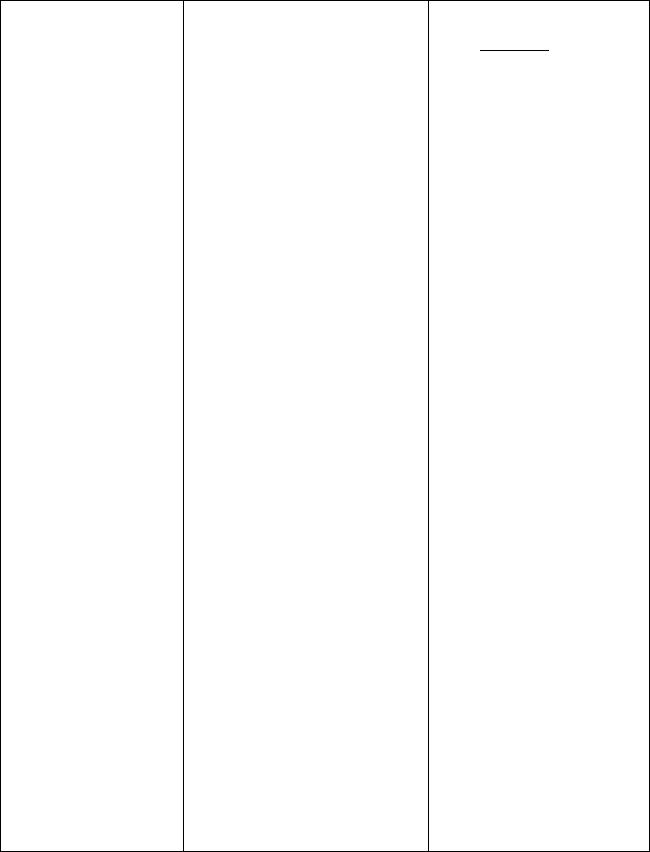WAEC Syllabus – Uploaded online by www.myschoolgist.com.ng

(ii) The derivative of a function

(iii)Differentiation of polynomials

(iv)Differentiation of trigonometric Functions

(v)Product and quotient rules. Differentiation of implicit

functions such as ax+ by= c

**(vi) Differentiation of Transcendental Functions

(vii)Second order derivatives and Rates of change and small changes (x), Concept of Maxima and Minima

(16)Integration

(i) Indefinite Integral

Relate to the gradient of a curve. e.g. f1(x) =

limC→* (C ) ().

C

(ii)Its meaning and its determination from first

principles (simple cases only).

e.g. ax+ b, n ≤ 3, (n )

e.g. ax– bxm – 1+ …+k, where m Є , k is a constant.

e.g. sin x, y = a sin x ± b cos x. Where a, b are constants.

including polynomials of the form (a + bxn)m.

e.g. y = eax, y = log 3x, y = ln x

(i)The equation of a tangent to a curve at a point.

(ii)Restrict turning points to maxima and minima.

(iii)Include curve sketching (up to cubic functions) and linear

kinematics.

(i) Integration of polynomials of the form axn; n ≠ -1. i.e.

 ∫xn dx = E> + c, n ≠ -1. (

(ii)Integration of sum and

difference of polynomials. e.g. ∫(4x3+3x2-6x+5) dx

**(iii)Integration of polynomials of the form axn; n = -1.WAEC Syllabus – Uploaded online by www.myschoolgist.com.ng

II. Statistics and

Probability

(17)Statistics

(ii)Definite Integral

(iii)Applications of the Definite Integral

(i)Tabulation and Graphical representation of data

(ii)Measures of location

(iii) Measures of Dispersion

i.e. ∫ -1 dx = ln x

Simple problems on integration by substitution.

Integration of simple trigonometric functions of the

form Fsin G .G.

(i) Plane areas and Rate of Change. Include linear

kinematics.

Relate to the area under a curve.

(ii)Volume of solid of revolution

(iii)Approximation restricted to trapezium rule.

Frequency tables. Cumulative frequency tables. Histogram (including unequal class intervals).

Cumulative frequency curve (Ogive) for grouped data.

Central tendency: mean, median, mode, quartiles and percentiles.

Mode and modal group for grouped data from a histogram. Median from grouped data. Mean for grouped data (use of an assumed mean required).

Determination of:

(i) Range, Inter- Quartile and Semi inter-quartilerange

from an Ogive.

(ii)Mean deviation, variance and standard deviation for grouped and ungroupedWAEC Syllabus – Uploaded online by www.myschoolgist.com.ng

(iv)Correlation

(18)Probability

(i)Meaning of probability.

(ii)Relative frequency.

(iii)Calculation of Probability using simple sample spaces.

(v)Probability distributions.

III. Vectors and

Mechanics

(19)Vectors

(i)Definitions of scalar and vector Quantities.

(ii)Representation of Vectors.

data. Using an assumed mean or true mean.

Scatter diagrams, use of line of best fit to predict one variable from another, meaning of correlation; positive, negative and zero correlations,. Spearman’s Rank coefficient. Use data without ties. *Equation of line of best fit by least square method. (Line of regression of y on x).

Tossing 2 dice once; drawing from a box with or without replacement.

Equally likely events, mutually exclusive, independent and conditional events.

Include the probability of an event considered as the probability of a set.

(i)Binomial distributionP(x=r)=nCrprqn-r ,where

Probability of success = p, Probability of failure = q,

p + q = 1 and n is the number of trials. Simple problems only.

**(ii) Poisson distribution

 P(x) = HIJK , where λ = np, !

n is large and p is small.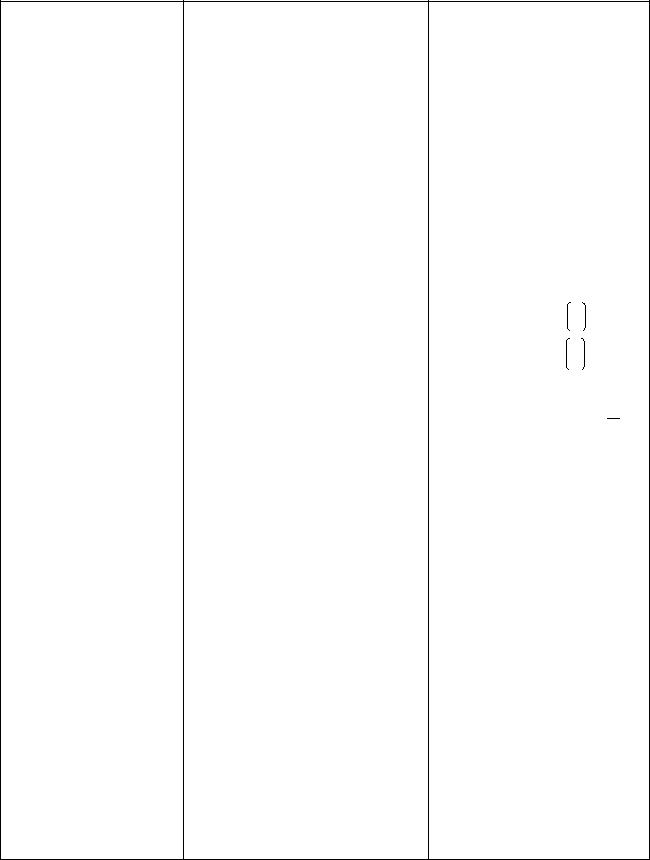WAEC Syllabus – Uploaded online by www.myschoolgist.com.ng

(iii)Algebra of Vectors.

(iv)Commutative, Associative and Distributive Properties.

(v)Unit vectors.

(vi) Position Vectors.

(vii)Resolution and Composition of Vectors.

(viii) Scalar (dot) product and its

Representation of vector )in the form a+ bj.

Addition and subtraction, multiplication of vectors by vectors, scalars and equation of vectors. Triangle, Parallelogram and polygon Laws.

Illustrate through diagram, Illustrate by solving problems in elementary plane geometry e.g con-currency of medians and diagonals.

The notation:

for the unit vector 1 and 0

for the unit vector 0 1 along the x and y axes

respectively. Calculation of unit vector (â) along a i.e. â = ||.

Position vector of A relative to

Ois PPPPPQNO.

Position vector of the midpoint of a line segment. Relate to coordinates of mid-point of a line segment.

*Position vector of a point that divides a line segment internally in the ratio (λ : μ).

Applying triangle, parallelogram and polygon laws to composition of forces acting at a point. e.g. find the resultant of two forces (12N, 030o) and (8N, 100o) acting at a point.

*Find the resultant of vectors by scale drawing.

Finding angle between two vectors.

Using the dot product to application.

**(ix) Vector (cross) product and its application.

(20)Statics

(i)Definition of a force.

(ii)Representation of forces.

(iii)Composition and resolution of coplanar forces acting at a

point.

(iv)Composition and resolution of general coplanar forces on

rigid bodies.

(v)Equilibrium of Bodies.

(vi)Determination of Resultant.

(vii)Moments of forces.

(viii)Friction.

(21)Dynamics

establish such trigonometric formulae as

(i) Cos (a ± b) =

cos a cos b ∓ sin a sin b

(ii) sin (a ± b)=

sin a cos b ± sin cosa

(iii)c= a+ b– 2ab cos C

(iv)STU=STUSTU  Y.

Apply to simple problems e.g. suspension of particles by strings.

Resultant of forces, Lami’s theorem

Using the principles of moments to solve related problems.

Distinction between smooth and rough planes. Determination of the coefficient of friction.

WAEC Syllabus – Uploaded online by www.myschoolgist.com.ng

(i)The concepts of motion

(ii)Equations of Motion

(iii)The impulse and momentum equations:

**(iv) Projectiles.

The definitions of displacement, velocity, acceleration and speed.

Composition of velocities and accelerations.

Rectilinear motion. Newton’s laws of motion. Application of Newton’s Laws Motion along inclined planes (resolving a force upon a plane into normal and frictional forces).

Motion under gravity (ignore air resistance).

Application of the equations of

motions: V = u + at, S = ut + ½ at 2; v= u+ 2as.

Conservation of Linear Momentum(exclude coefficient of restitution).

Distinguish between momentum and impulse.

Objects projected at an angle to the horizontal.

1.UNITS

Candidates should be familiar with the following units and their symbols.

(1 ) Length

1000 millimetres (mm) = 100 centimetres (cm) = 1 metre(m). 1000 metres = 1 kilometre (km)

(2 ) Area

10,000 square metres (m2) = 1 hectare (ha)

( 3 ) CapacityWAEC Syllabus – Uploaded online by www.myschoolgist.com.ng

1000 cubic centimeters (cm3) = 1 litre (l)

(4 ) Mass

1000milligrammes (mg) = 1 gramme (g)

1000 grammes (g) = 1 kilogramme( kg )

1000 ogrammes (kg) = 1 tonne.

 ( 5) Currencies The Gambia – 100 bututs (b) = 1 Dalasi (D) Ghana – 100 Ghana pesewas (Gp) = 1 Ghana Cedi ( GH¢) Liberia – 100 cents (c) = 1 Liberian Dollar (LD) Nigeria – 100 kobo (k) = 1 Naira ( N ) Sierra Leone – 100 cents (c) = 1 Leone (Le) UK – 100 pence (p) = 1 pound (£) USA – 100 cents (c) = 1 dollar (\$) French Speaking territories 100 centimes (c) = 1 Franc (fr)

Any other units used will be defined.

2.OTHER IMPORTANT INFORMATION

( 1) Use of Mathematical and Statistical Tables

Mathematics and Statistical tables, published or approved by WAEC may be used in the examination room. Where the degree of accuracy is not specified in a question, the degree of accuracy expected will be that obtainable from the mathematical tables.

(2)Use of calculators

The use of non-programmable, silent and cordless calculators is allowed. The calculators must, however not have a paper print out nor be capable of receiving/sending any information. Phones with or without calculators are not allowed.

(3)Other Materials Required for the examination

Candidates should bring rulers, pairs of compasses, protractors, set squares etc required for papers of the subject. They will not be allowed to borrow such instruments and any other material from other candidates in the examination hall.

Graph papers ruled in 2mm squares will be provided for any paper in which it is required.

( 4) Disclaimer

In spite of the provisions made in paragraphs 2 (1) and (2) above, it should be noted that some questions may prohibit the use of tables and/or calculators.